Type
Quiz
Book Title
Fundamentals of Corporate Finance Standard Edition 9th Edition
ISBN 13
978-0073382395

### 978-0073382395 Chapter 4 Questions and Problems 10-20

April 3, 2019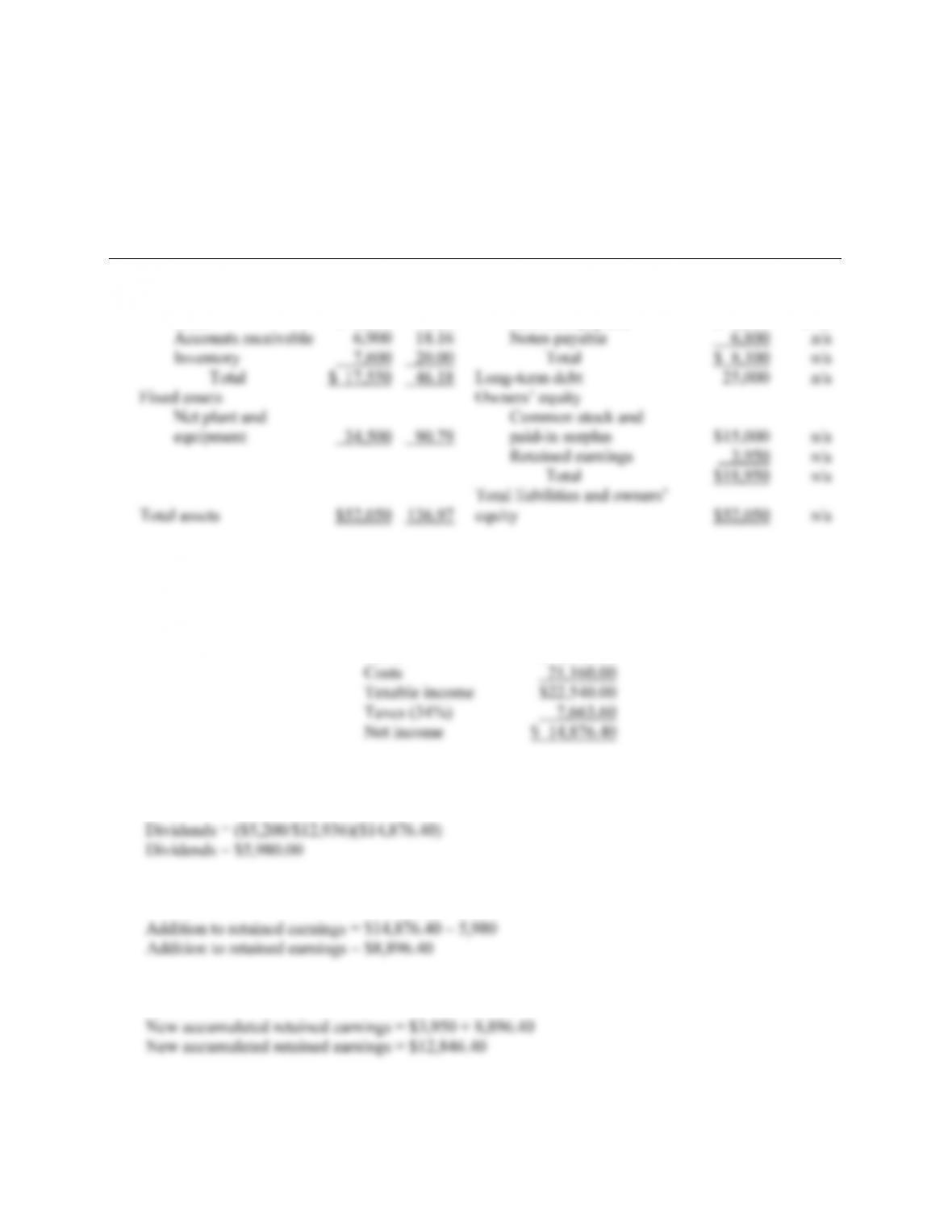CHAPTER 4 B-35
10. Below is the balance sheet with the percentage of sales for each account on the balance sheet. Notes
payable, total current liabilities, long-term debt, and all equity accounts do not vary directly with
sales.
HEIR JORDAN CORPORATION
Balance Sheet
(\$) (%) (\$) (%)
Assets Liabilities and Owners’ Equity
Current assets Current liabilities
Cash \$ 3,050 8.03 Accounts payable \$ 1,300 3.42
11. Assuming costs vary with sales and a 15 percent increase in sales, the pro forma income statement
will look like this:
HEIR JORDAN CORPORATION
Pro Forma Income Statement
Sales \$43,700.00
The payout ratio is constant, so the dividends paid this year is the payout ratio from last year times
net income, or:
And the addition to retained earnings will be:
The new accumulated retained earnings on the pro forma balance sheet will be: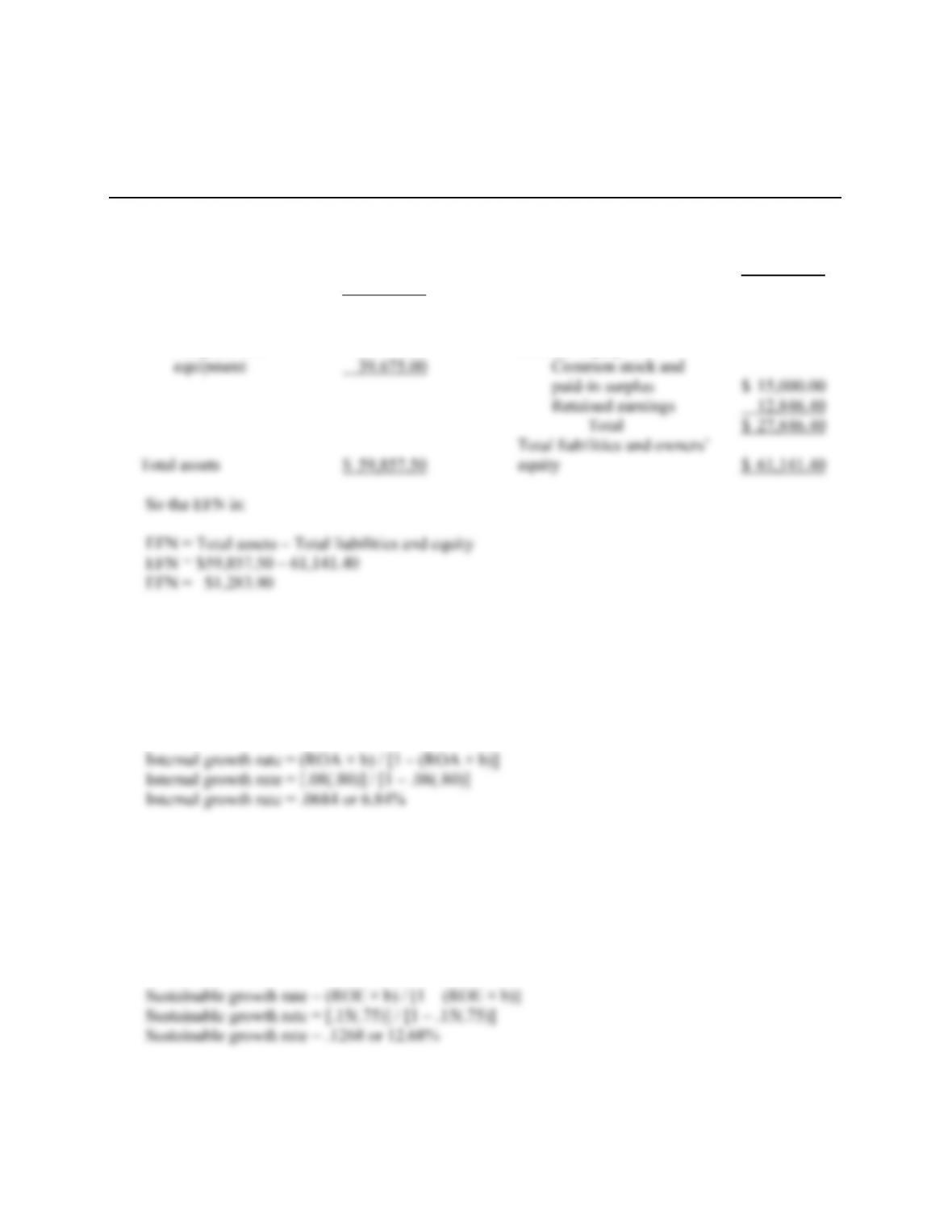B-36 SOLUTIONS
The pro forma balance sheet will look like this:
HEIR JORDAN CORPORATION
Pro Forma Balance Sheet
Assets Liabilities and Owners’ Equity
Current assets Current liabilities
Cash \$ 3,507.50 Accounts payable \$ 1,495.00
Accounts receivable 7,935.00 Notes payable 6,800.00
Inventory 8,740.00 Total \$ 8,295.00
Total \$20,182.50 Long-term debt 25,000.00
Fixed assets
Net plant and Owners’ equity
12. We need to calculate the retention ratio to calculate the internal growth rate. The retention ratio is:
b = 1 – .20
b = .80
Now we can use the internal growth rate equation to get:
13. We need to calculate the retention ratio to calculate the sustainable growth rate. The retention ratio
is:
b = 1 – .25
b = .75
Now we can use the sustainable growth rate equation to get: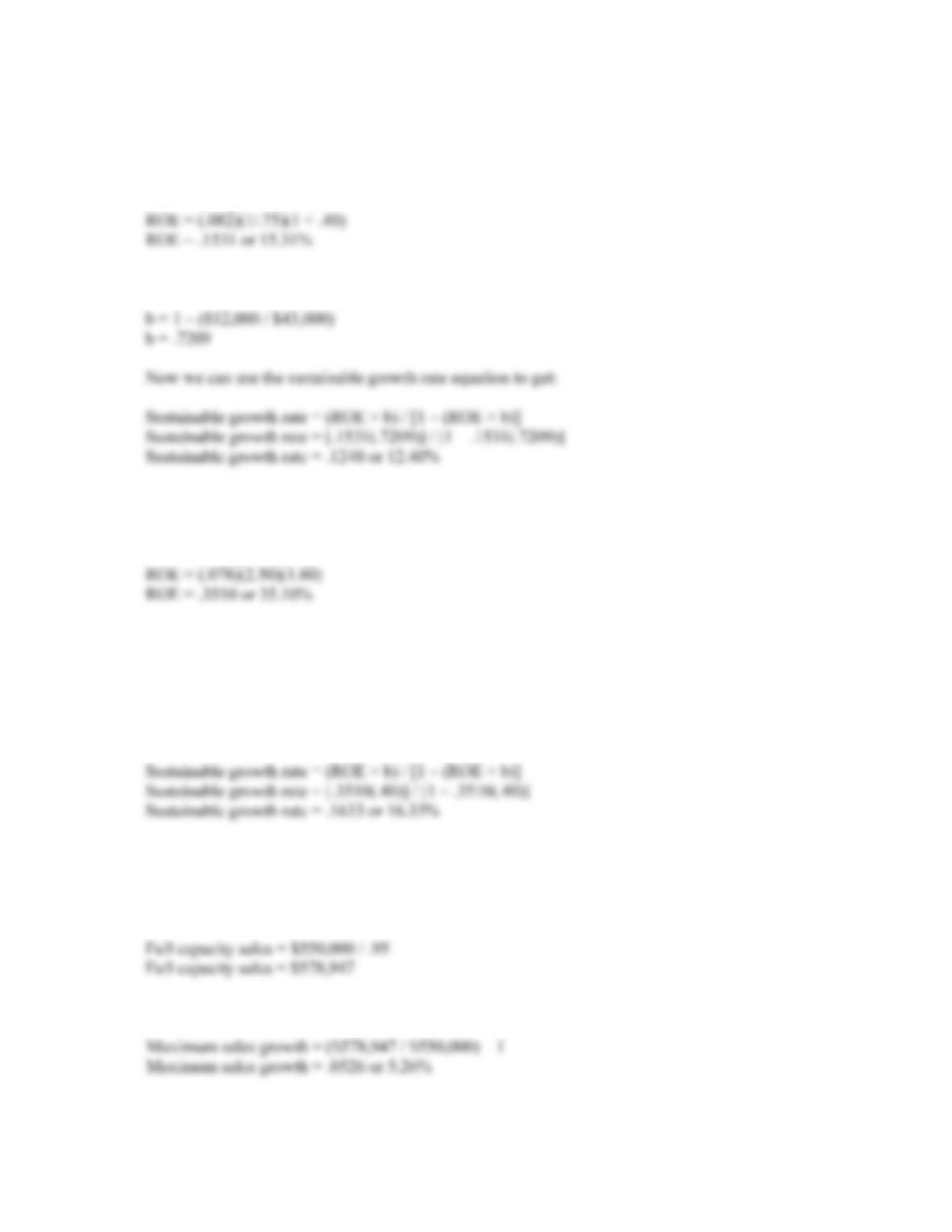CHAPTER 4 B-37
14. We first must calculate the ROE to calculate the sustainable growth rate. To do this we must realize
two other relationships. The total asset turnover is the inverse of the capital intensity ratio, and the
equity multiplier is 1 + D/E. Using these relationships, we get:
ROE = (PM)(TAT)(EM)
The plowback ratio is one minus the dividend payout ratio, so:
15. We must first calculate the ROE using the DuPont ratio to calculate the sustainable growth rate. The
ROE is:
ROE = (PM)(TAT)(EM)
The plowback ratio is one minus the dividend payout ratio, so:
b = 1 – .60
b = .40
Now we can use the sustainable growth rate equation to get:
Intermediate
16. To determine full capacity sales, we divide the current sales by the capacity the company is currently
using, so:
The maximum sales growth is the full capacity sales divided by the current sales, so: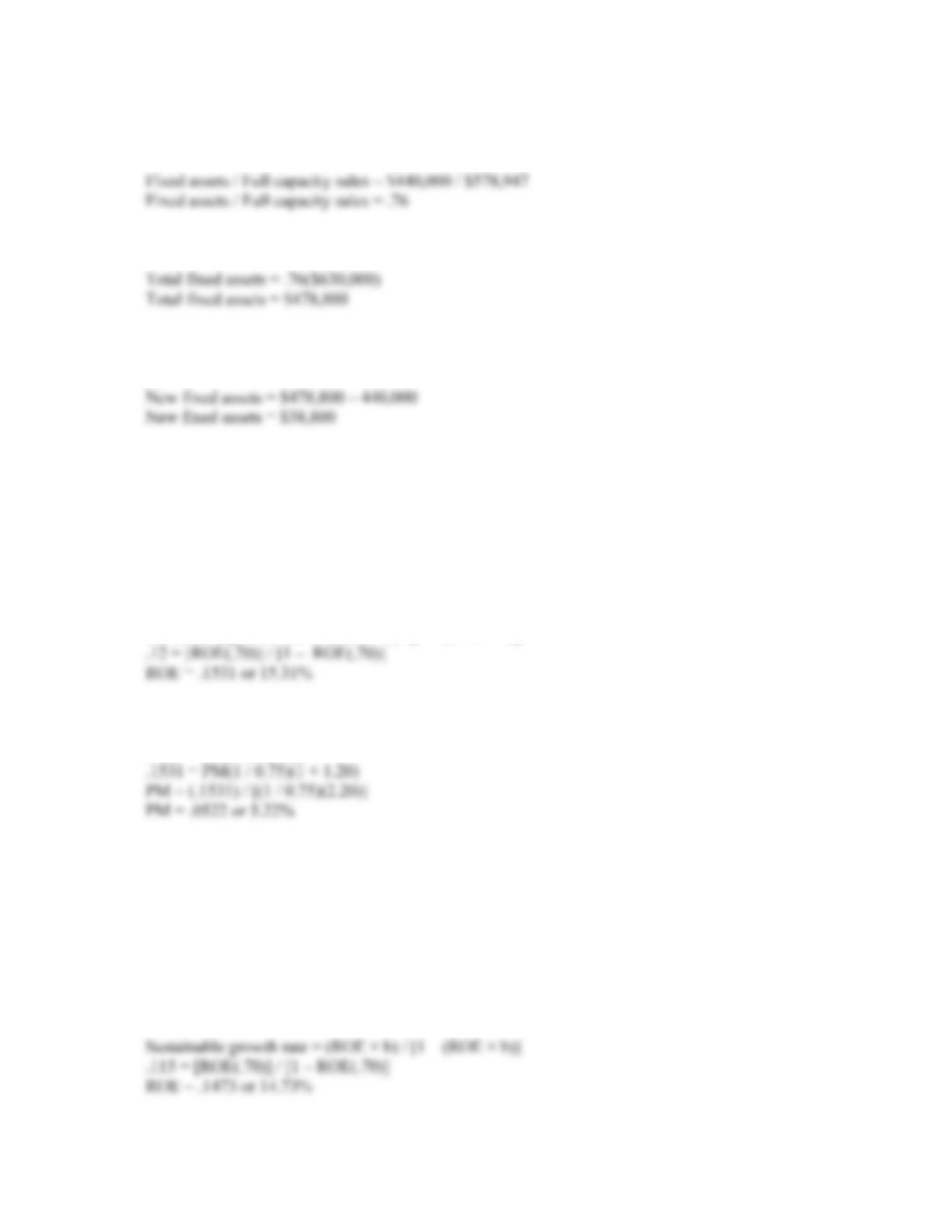B-38 SOLUTIONS
17. To find the new level of fixed assets, we need to find the current percentage of fixed assets to full
capacity sales. Doing so, we find:
Next, we calculate the total dollar amount of fixed assets needed at the new sales figure.
The new fixed assets necessary is the total fixed assets at the new sales figure minus the current level
of fixed assts.
18. We have all the variables to calculate ROE using the DuPont identity except the profit margin. If we
find ROE, we can solve the DuPont identity for profit margin. We can calculate ROE from the
sustainable growth rate equation. For this equation we need the retention ratio, so:
b = 1 – .30
b = .70
Using the sustainable growth rate equation and solving for ROE, we get:
Sustainable growth rate = (ROE × b) / [1 – (ROE × b)]
Now we can use the DuPont identity to find the profit margin as:
ROE = PM(TAT)(EM)
19. We have all the variables to calculate ROE using the DuPont identity except the equity multiplier.
Remember that the equity multiplier is one plus the debt-equity ratio. If we find ROE, we can solve
the DuPont identity for equity multiplier, then the debt-equity ratio. We can calculate ROE from the
sustainable growth rate equation. For this equation we need the retention ratio, so:
b = 1 – .30
b = .70
Using the sustainable growth rate equation and solving for ROE, we get: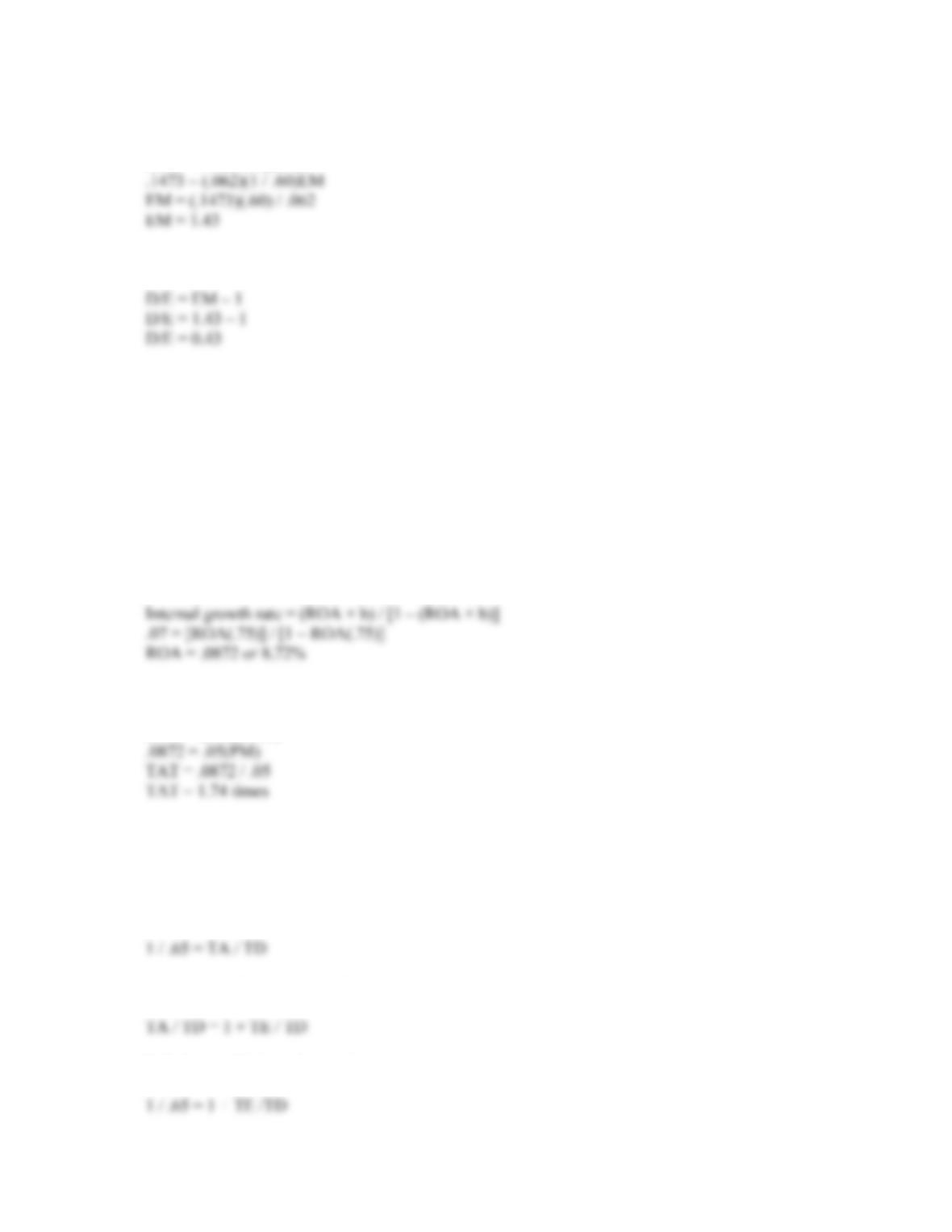CHAPTER 4 B-39
Now we can use the DuPont identity to find the equity multiplier as:
ROE = PM(TAT)(EM)
So, the D/E ratio is:
20. We are given the profit margin. Remember that:
ROA = PM(TAT)
We can calculate the ROA from the internal growth rate formula, and then use the ROA in this
equation to find the total asset turnover. The retention ratio is:
b = 1 – .25
b = .75
Using the internal growth rate equation to find the ROA, we get:
Plugging ROA and PM into the equation we began with and solving for TAT, we get:
ROA = (PM)(TAT)
21. We should begin by calculating the D/E ratio. We calculate the D/E ratio as follows:
Total debt ratio = .65 = TD / TA
Inverting both sides we get:
Next, we need to recognize that
Substituting this into the previous equation, we get: